Chapter 5 Class 12 Continuity and Differentiability

Class 12
Important Questions for exams Class 12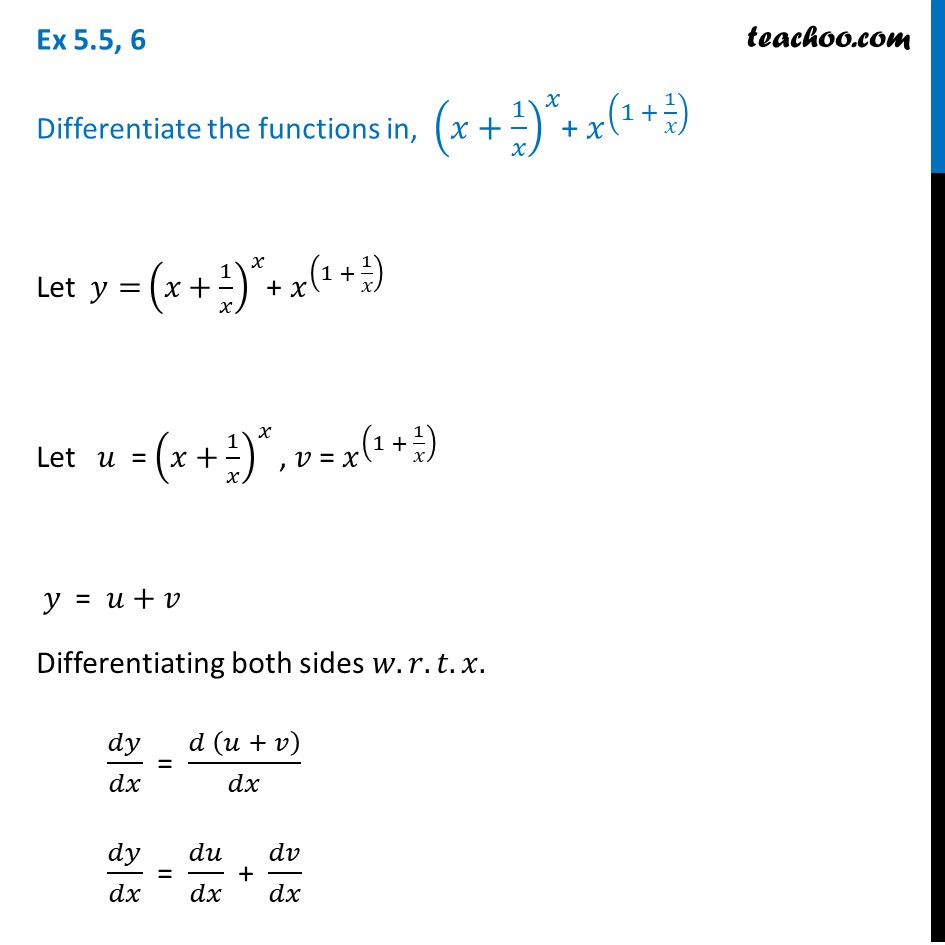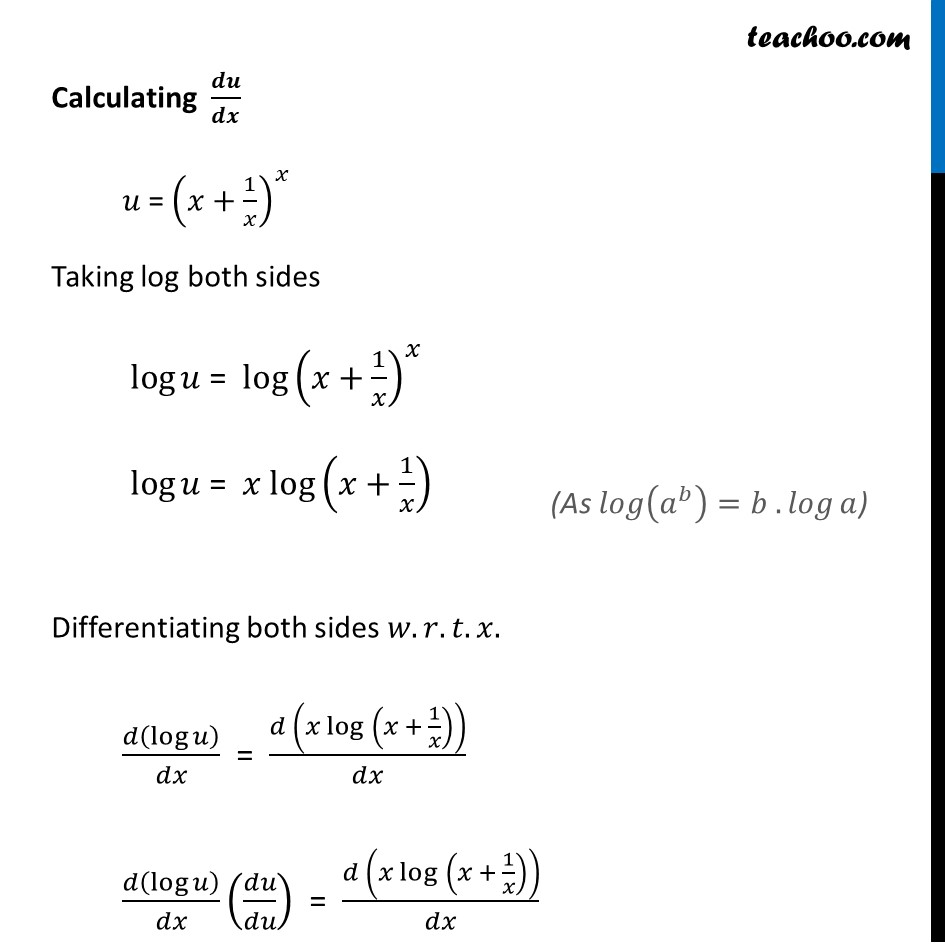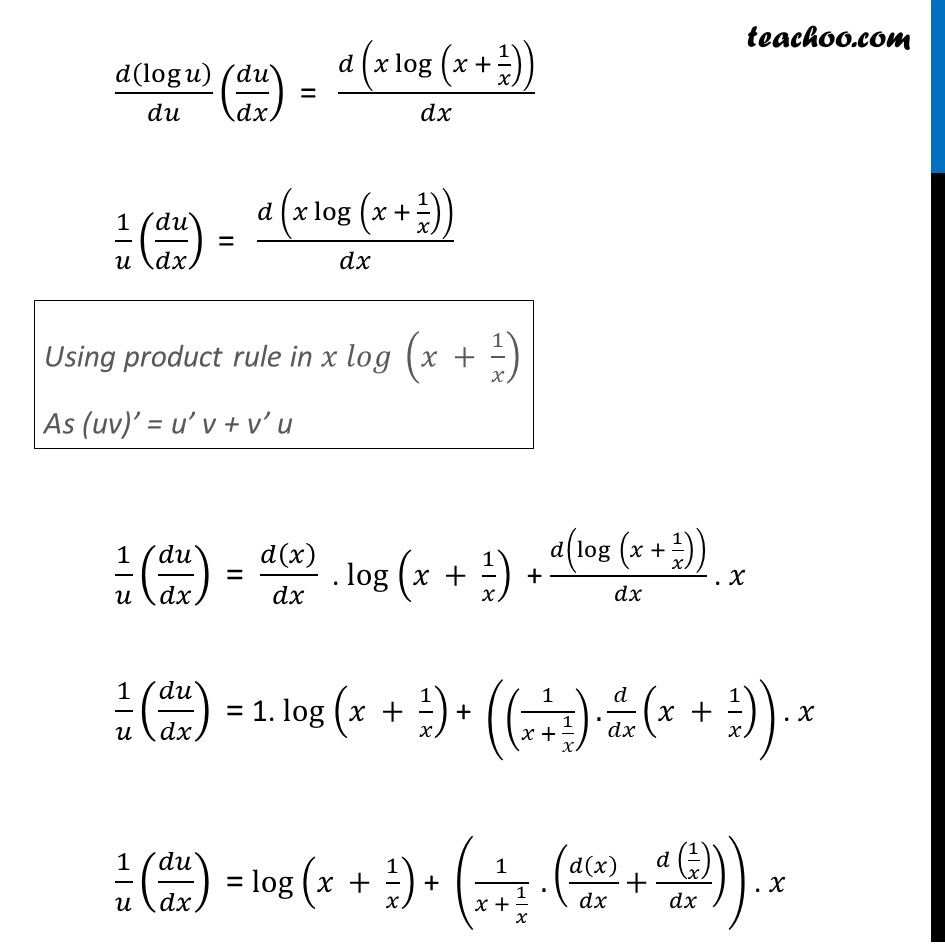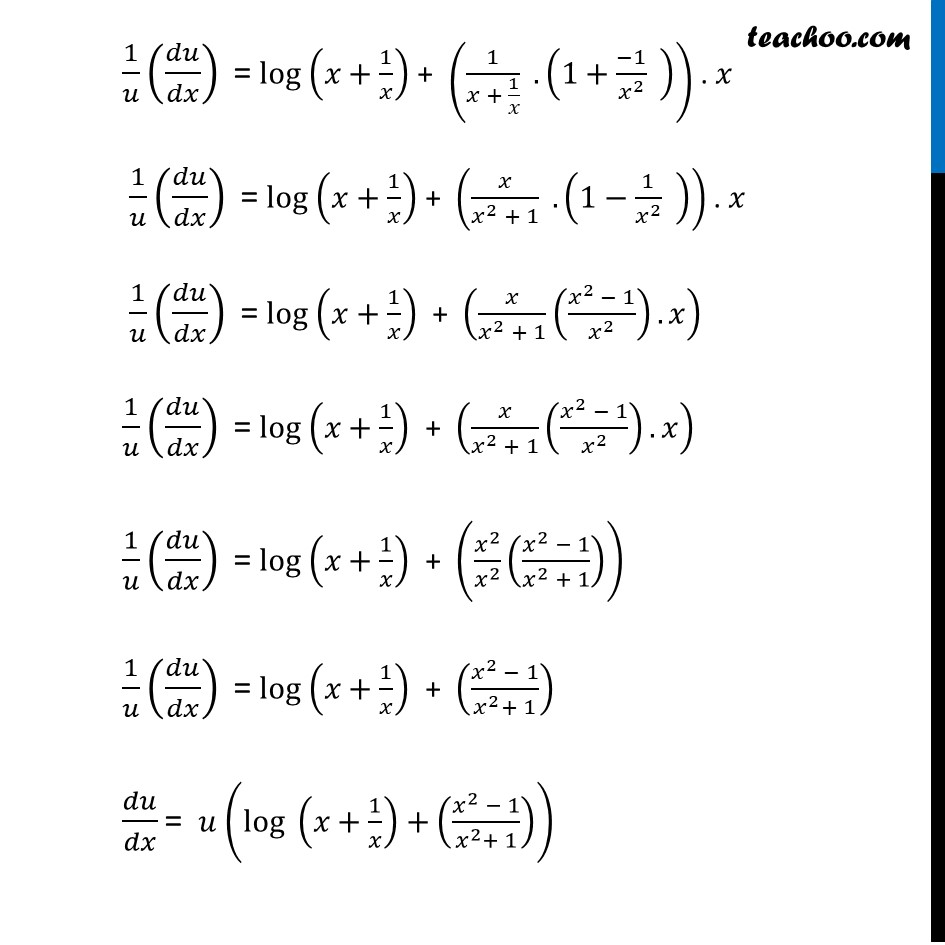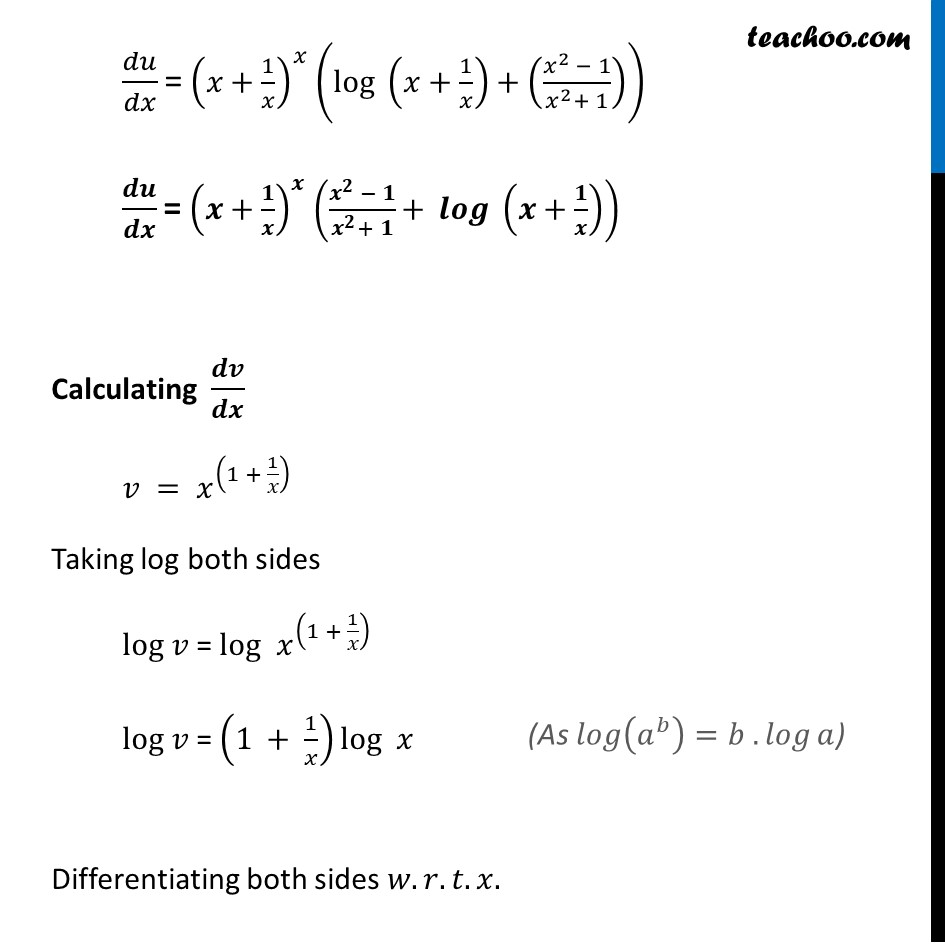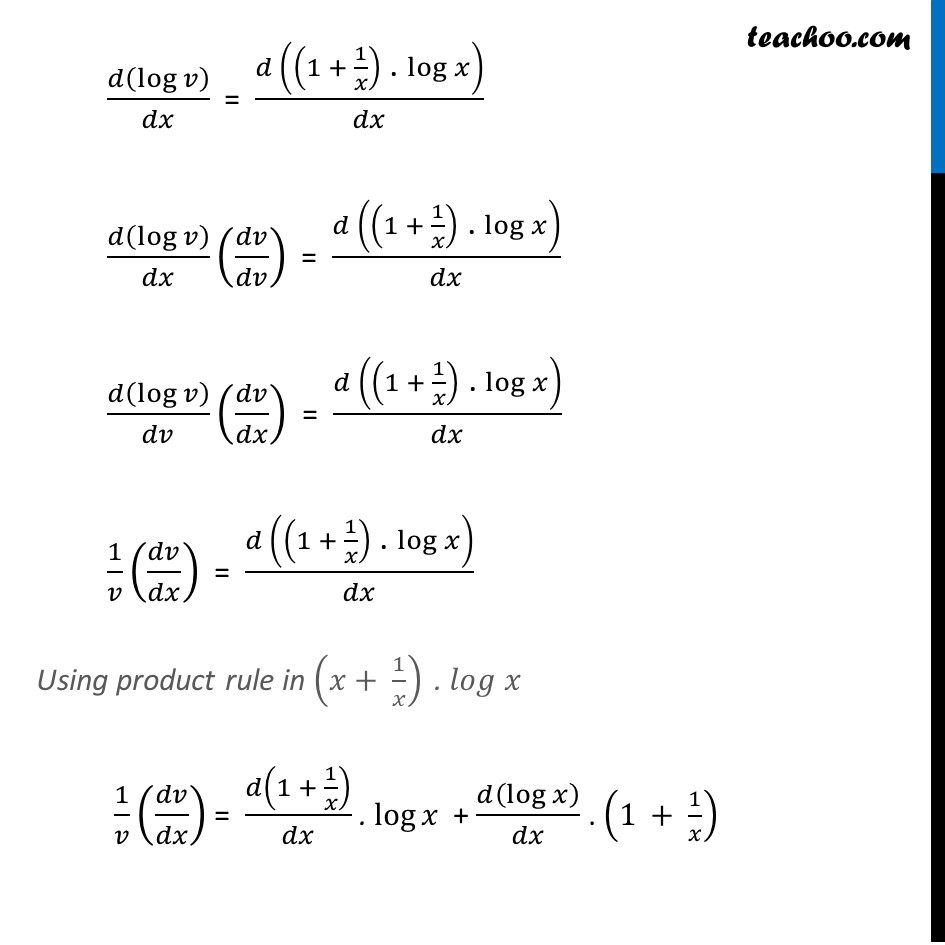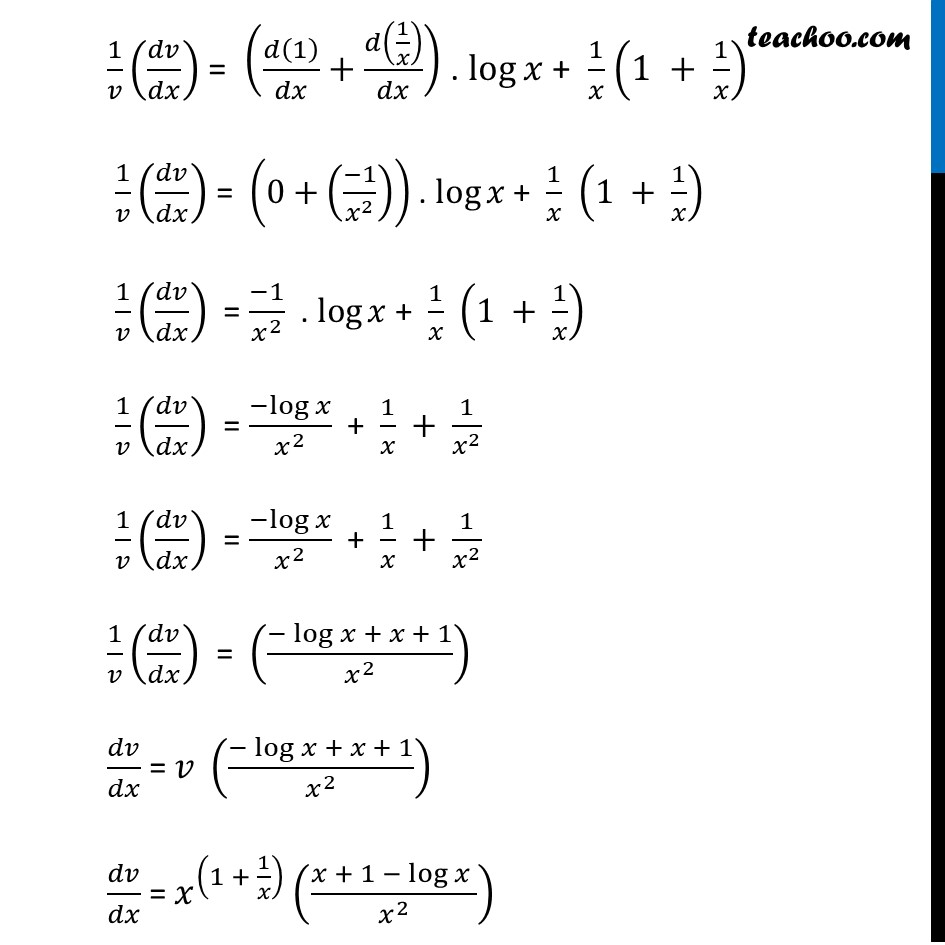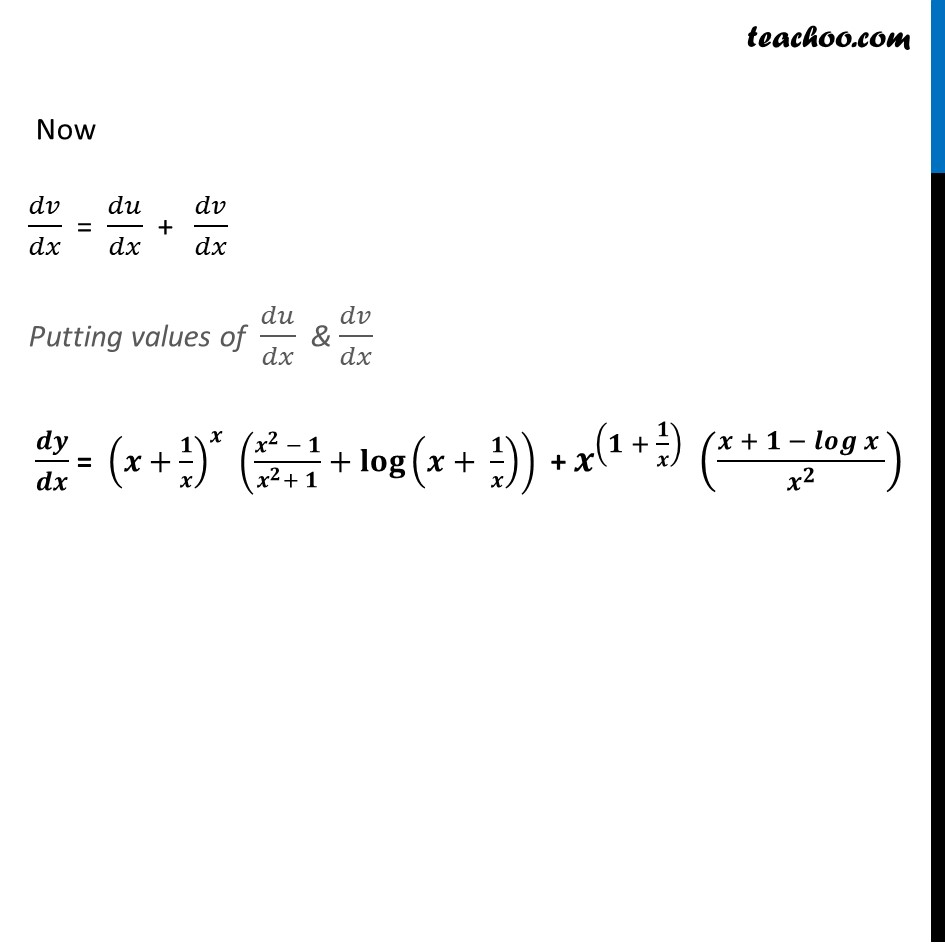Learn in your speed, with individual attention - Teachoo Maths 1-on-1 Class

### Transcript

Ex 5.5, 6 Differentiate the functions in, (𝑥+1/𝑥)^𝑥+ 𝑥^((1 + 1/𝑥) ) Let 𝑦= (𝑥+1/𝑥)^𝑥+ 𝑥^((1 + 1/𝑥) ) Let 𝑢 = (𝑥+1/𝑥)^𝑥 , 𝑣 = 𝑥^((1 + 1/𝑥) ) 𝑦 = 𝑢+𝑣 Differentiating both sides 𝑤.𝑟.𝑡.𝑥. 𝑑𝑦/𝑑𝑥 = (𝑑 (𝑢 + 𝑣))/𝑑𝑥 𝑑𝑦/𝑑𝑥 = 𝑑𝑢/𝑑𝑥 + 𝑑𝑣/𝑑𝑥 Calculating 𝒅𝒖/𝒅𝒙 𝑢 = (𝑥+1/𝑥)^𝑥 Taking log both sides log⁡𝑢 = log (𝑥+1/𝑥)^𝑥 log⁡𝑢 = 𝑥 log (𝑥+1/𝑥) Differentiating both sides 𝑤.𝑟.𝑡.𝑥. 𝑑(log⁡𝑢 )/𝑑𝑥 = (𝑑 (𝑥 log" " (𝑥 + 1/𝑥)))/𝑑𝑥 𝑑(log⁡𝑢 )/𝑑𝑥 (𝑑𝑢/𝑑𝑢) = (𝑑 (𝑥 log" " (𝑥 + 1/𝑥)))/𝑑𝑥 (As 𝑙𝑜𝑔⁡(𝑎^𝑏 )=𝑏 . 𝑙𝑜𝑔⁡𝑎) 𝑑(log⁡𝑢 )/𝑑𝑢 (𝑑𝑢/𝑑𝑥)" = " (𝑑 (𝑥 log" " (𝑥 + 1/𝑥)))/𝑑𝑥 1/𝑢 (𝑑𝑢/𝑑𝑥)" = " (𝑑 (𝑥 log" " (𝑥 + 1/𝑥)))/𝑑𝑥 1/𝑢 (𝑑𝑢/𝑑𝑥)" = " 𝑑(𝑥)/𝑑𝑥 . log (𝑥 + 1/𝑥) + 𝑑(log" " (𝑥 + 1/𝑥))/𝑑𝑥 . 𝑥 1/𝑢 (𝑑𝑢/𝑑𝑥)" =" 1. log (𝑥 + 1/𝑥) + ((1/(𝑥 + 1/𝑥)).𝑑/𝑑𝑥 (𝑥 + 1/𝑥)) . 𝑥 1/𝑢 (𝑑𝑢/𝑑𝑥)" =" log (𝑥 + 1/𝑥) + (1/(𝑥 + 1/𝑥) . (𝑑(𝑥)/𝑑𝑥+(𝑑 (1/𝑥))/𝑑𝑥)) . 𝑥 Using product rule in 𝑥 𝑙𝑜𝑔" " (𝑥 + 1/𝑥) As (uv)’ = u’ v + v’ u 1/𝑢 (𝑑𝑢/𝑑𝑥)" =" log (𝑥+1/𝑥) + (1/(𝑥 + 1/𝑥) . (1+(−1)/𝑥^2 " " )) . 𝑥 1/𝑢 (𝑑𝑢/𝑑𝑥)" =" log (𝑥+1/𝑥) + (𝑥/(𝑥^2 + 1) . (1−1/𝑥^2 " " )) . 𝑥 1/𝑢 (𝑑𝑢/𝑑𝑥)" =" log (𝑥+1/𝑥) + (𝑥/(𝑥^2 + 1) ((𝑥^2 − 1)/𝑥^2 ).𝑥) 1/𝑢 (𝑑𝑢/𝑑𝑥)" =" log (𝑥+1/𝑥) + (𝑥/(𝑥^2 + 1) ((𝑥^2 − 1)/𝑥^2 ).𝑥) 1/𝑢 (𝑑𝑢/𝑑𝑥)" =" log (𝑥+1/𝑥) + (𝑥^2/𝑥^2 ((𝑥^2 − 1)/(𝑥^2 + 1))) 1/𝑢 (𝑑𝑢/𝑑𝑥)" =" log (𝑥+1/𝑥) + ((𝑥^2 − 1)/(𝑥^2+ 1)) 𝑑𝑢/𝑑𝑥 "= " 𝑢 (〖log 〗⁡(𝑥+1/𝑥)+((𝑥^2 − 1)/(𝑥^2+ 1))) 𝑑𝑢/𝑑𝑥 "=" (𝑥+1/𝑥)^𝑥 (〖log 〗⁡(𝑥+1/𝑥)+((𝑥^2 − 1)/(𝑥^2+ 1))) 𝒅𝒖/𝒅𝒙 "=" (𝒙+𝟏/𝒙)^𝒙 ((𝒙^𝟐 − 𝟏)/(𝒙^𝟐+ 𝟏)⁡〖+ 〖𝒍𝒐𝒈 〗⁡(𝒙+𝟏/𝒙) 〗 ) Calculating 𝒅𝒗/𝒅𝒙 𝑣 = 𝑥^(1 + 1/𝑥)" " Taking log both sides log 𝑣 = log 𝑥^(1 + 1/𝑥)" " log 𝑣 = (1 + 1/𝑥)log 𝑥^" " Differentiating both sides 𝑤.𝑟.𝑡.𝑥. 𝑑(log⁡𝑣 )/𝑑𝑥 = (𝑑 ((1 + 1/𝑥)" . " log 𝑥))/𝑑𝑥 𝑑(log⁡𝑣 )/𝑑𝑥 (𝑑𝑣/𝑑𝑣) = (𝑑 ((1 + 1/𝑥)" . " log 𝑥))/𝑑𝑥 𝑑(log⁡𝑣 )/𝑑𝑣 (𝑑𝑣/𝑑𝑥) = (𝑑 ((1 + 1/𝑥)" . " log 𝑥))/𝑑𝑥 1/𝑣 (𝑑𝑣/𝑑𝑥) = (𝑑 ((1 + 1/𝑥)" . " log 𝑥))/𝑑𝑥 Using product rule in (𝑥+ 1/𝑥)" . " 𝑙𝑜𝑔 𝑥 1/𝑣 (𝑑𝑣/𝑑𝑥) = 𝑑(1 + 1/𝑥)/𝑑𝑥 . log⁡𝑥 + 𝑑(log⁡𝑥 )/𝑑𝑥 . (1 + 1/𝑥) 1/𝑣 (𝑑𝑣/𝑑𝑥) = (𝑑(1)/𝑑𝑥+𝑑(1/𝑥)/𝑑𝑥) . log⁡𝑥 + 1/𝑥 (1 + 1/𝑥) 1/𝑣 (𝑑𝑣/𝑑𝑥) = (0+((−1)/𝑥^2 )) . log⁡𝑥 + 1/𝑥 (1 + 1/𝑥) 1/𝑣 (𝑑𝑣/𝑑𝑥) = (−1)/𝑥^2 . log⁡𝑥 + 1/𝑥 (1 + 1/𝑥) 1/𝑣 (𝑑𝑣/𝑑𝑥) = (−log⁡𝑥)/𝑥^2 + 1/𝑥 + 1/𝑥^2 1/𝑣 (𝑑𝑣/𝑑𝑥) = (−log⁡𝑥)/𝑥^2 + 1/𝑥 + 1/𝑥^2 1/𝑣 (𝑑𝑣/𝑑𝑥) = ((−log⁡𝑥 + 𝑥 + 1)/𝑥^2 ) 𝑑𝑣/𝑑𝑥 = 𝑣 ((−log⁡𝑥 + 𝑥 + 1)/𝑥^2 ) 𝑑𝑣/𝑑𝑥 = 𝑥^((1 + 1/𝑥) ) ((𝑥 + 1 − log⁡𝑥 )/𝑥^2 ) Now 𝑑𝑣/𝑑𝑥 = 𝑑𝑢/𝑑𝑥 + 𝑑𝑣/𝑑𝑥 Putting values of 𝑑𝑢/𝑑𝑥 & 𝑑𝑣/𝑑𝑥 𝒅𝒚/𝒅𝒙 = (𝒙+𝟏/𝒙)^𝒙 ((𝒙^𝟐 − 𝟏)/(𝒙^𝟐+ 𝟏)+𝐥𝐨𝐠⁡(𝒙+ 𝟏/𝒙) ) + 𝒙^((𝟏 + 𝟏/𝒙) ) ((𝒙 + 𝟏 − 𝒍𝒐𝒈⁡𝒙 )/𝒙^𝟐 )